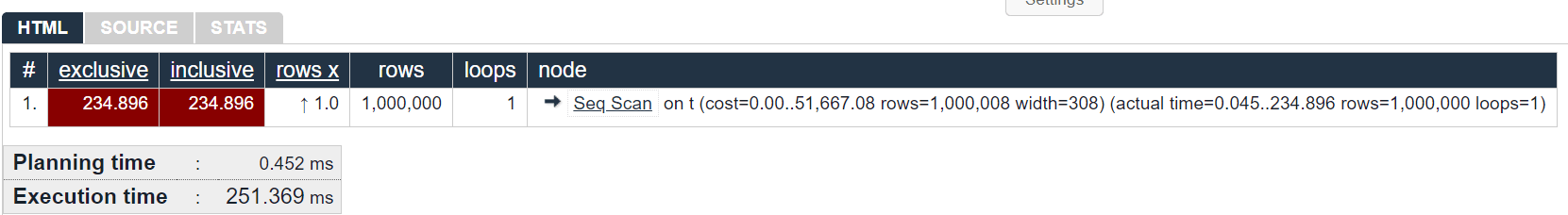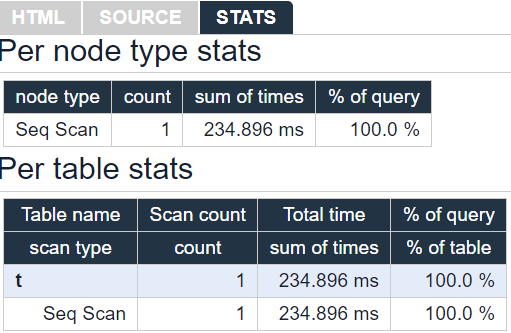# PostgreSQL – Explaining the EXPLAIN Command

#### Introduction

PostgreSQL is undeniably one of the best DBMSs. It’s open-source and community-driven, which has helped increase its popularity over the years. As of February 2020, PostgreSQL is placed on the 4th place by popularity, falling behind only Microsoft SQL Server, MySQL, and Oracle.

PostgreSQL has many tools which the developer can make use of, and one such tool is the EXPLAIN command. The EXPLAIN command returns the execution plan, which the PostgreSQL planner creates for a given statement.

##### So, what is this planner and how does it work?

The first thing that you need to understand is that PostgreSQL keeps some metadata – information about information, like the number of rows from tables, most common values, number of distinct values, etc. PostgreSQL keeps track of all this data.

Before PostgreSQL executes a statement, it first builds an execution plan. This is done to ensure that PostgreSQL fetches the data we need, in the fastest and most optimal way.

The plan is influenced by many things, but the most important factors are the cost of the operations (we will analyze this more thoroughly later), and the metadata.

#### The EXPLAIN command

This is what the syntax of the EXPLAIN command looks like:

```EXPLAIN [ ( option [, ...] ) ] sql_statement;

```

Where option can be:

```ANALYZE [ boolean ] – By default this is TRUE
VERBOSE [ boolean ] – By default this is FALSE
COSTS [ boolean ] – By default this is TRUE
TIMING [ boolean ] - By default this is TRUE, can only be used with ANALYZE
SUMMARY [ boolean ] – By default, FALSE if ANALYZE is not used, TRUE otherwise
BUFFERS [ boolean ] - By default this is FALSE, can only be used with ANALYZE
FORMAT { TEXT | XML | JSON | YAML } – By default this is TEXT

```

We won’t analyze all of these options, however, in terms of usefulness, here are the commands that you should focus on the most: ANALYZE, COSTS and TIMING.

Now, let’s a take a look at the output of the EXPLAIN command:

```explain select * from test where id = 3;

```
And the output:
```                               QUERY PLAN
-----------------------------------------------------------------------------
Seq Scan on test  (cost=0.00..25.88 rows=6 width=36)
Filter: (id = 3)

```

In this example, the first line tells us that a sequence scan operation was executed on the table test.

##### Seq Scan

Seq Scan means that PostgreSQL will read the whole table from top to bottom and return as many rows as we need, and we also have additional info about the Filter bellow. That tells us that PostgreSQL filtered the records during the sequence scan, in this case, by id with the value 3. It’s remarkably simple: if you have a Where clause in your query, you will always have Filter in your plan for any kind of scans.

Now, let’s talk about the rest of the info that we can see in our example query plan. In the parenthesis next to the operation, you can see that we have 3 more pieces of information: costs, rows, and width.

##### Costs

Costs are the value measured on an arbitrary scale and represent how much it takes to ‘fetch a single page sequentially’. Costs are not estimated in seconds, but rather in resource usage. From the example above, we can see the following structure: number..number, where the first number shows the value of starting an operation, and the second number shows the expense of getting all rows returned by a given operation (the total cost of executing the operation).

The cost of starting an operation means how costly it is to even begin returning rows. For example, in the case of a Seq Scan, there is no starting cost. It just reads the data and immediately returns it. But in case we want to sort the data returned, the starting cost will quickly go up. That is because PostgreSQL has to first read all the data and sort it before returning the rows.

This can be seen in the following example:

```                               QUERY PLAN
-----------------------------------------------------------------------------
Sort  (cost=22.88..23.61 ROWS=292 width=202)
Sort KEY: relfilenode
->  Seq Scan ON pg_class  (cost=0.00..10.92 ROWS=292 width=202)

```

Notice that the total cost of the sorting operation is 23.61, while the cost of starting the operation is 22.88. This means that sorting the rows is expensive while returning them is trivial (in terms of costs).

In the postgres.conf file, we can find the following parameters:

```seq_page_cost = 1.0
random_page_cost = 4.0
cpu_tuple_cost = 0.01
cpu_index_tuple_cost = 0.005
cpu_operator_cost = 0.0025

```

All of these parameters dictate how costly it would be running different operations.

##### Rows

Rows is an estimated value of how many rows PostgreSQL thinks can return from a given operation. There are many ways how this number can be misestimated.

##### Width

This another estimated value of how many bytes, on average, PostgreSQL thinks would take a single row returned by a given operation.

Let’s take another example:

```CREATE TABLE test (id serial PRIMARY KEY, some_text text);
INSERT INTO test (some_text) SELECT 'testing' FROM generate_series(1,1000);

```

Now let’s run the EXPLAIN command with a condition on the id column:

```EXPLAIN SELECT * FROM test WHERE id = 50;

```
```                               QUERY PLAN
-----------------------------------------------------------------------------
Index Scan using test_pkey on test  (cost=0.28..8.29 rows=1 width=12)
Index Cond: (id = 50)

```

Things are different from the previous example. PostgreSQL uses an “INDEX Scan” this time.

##### INDEX Scan

Index scan means that PostgreSQL will read the page from the index, then check if there is a matching row in the table, and then read the page from the table. It may sound like twice the work when compared to a sequence scan, but in reality, index scans can speed up things by a lot. Index scans, however, are not always faster than sequence scans.

#### INDEX Scan vs Seq Scan

You might notice that sometimes, PostgreSQL still does a sequence scan on a table even if you have an index on it. Why is that?

Take a look at the following table:

```   COLUMN    |  TYPE   |                   Modifiers
-------------+---------+-----------------------------------------------------
id          | INTEGER | NOT NULL DEFAULT NEXTVAL('test_id_seq'::regclass)
test_column | INTEGER |
some_text   | text    |
Indexes:
"test_pkey" PRIMARY KEY, btree (id)
"q" btree (test_column)

```

And if we execute the following query:

```SELECT * FROM test WHERE some_column = 1;

```

Which one do you think PostgreSQL will choose? Index scan, or sequence scan? The answer is it depends.

If the table has only one row, and it has test_column = 1, then a sequence scan will be much faster because an index scan would take twice the effort, and PostgreSQL is smart enough to know that.

The same thing applies to big tables. If this table has 1 billion rows, and all of them have test_column = 1, then an index scan will be much, much slower than a sequence scan.

However, if this table has 1 billion rows, and only one of them has test_column = 1, then an index scan will be a lot faster compared to a sequence scan.

#### The ANALYZE option

One of the most commonly used options for the EXPLAIN command is the ANALYZE option. And that is for good reason because the ANALYZE option shows additional info in the execution plan about the real-time and costs it took for a query to execute.

Let’s take this example:

```CREATE TABLE t AS
SELECT i AS id, repeat('ISD', 100)::text AS payload
FROM generate_series(1, 1000000) i;

EXPLAIN analyze SELECT * FROM t;

```

The output is:

```                               QUERY PLAN
-----------------------------------------------------------------------------
Seq Scan on t  (cost=0.00..51667.08 rows=1000008 width=308)
(actual time=0.045..234.896 rows=1000000 loops=1)
Planning Time: 0.452 ms
Execution Time: 251.369 ms

```

We can immediately notice that there is more info. The first bit of additional information is another parenthesis on the Seq Scan operation. In the Seq, we see the actual time, the rows, and the loops, but bellow, appear Planning Time and Execution Time

##### Actual time

The actual time is a value which shows how costly was the execution of a given operation, with the same structure as the cost, but, it shows the actual costs (on average, because the same operation can run multiple times), not just estimates.

##### Rows

Rows show how many rows were returned by executing a given operation. Again, unlike rows from the first parenthesis, which are just estimates, this one shows how many rows were returned by the operation.

##### Loops

This is a new one. Loops show how many times a given operation was executed.

##### Planning Time

As mentioned at the beginning of this article, before executing a statement, PostgreSQL builds a plan. During the planning stage, PostgreSQL examines almost all possible execution plans, which, of course, takes time. Planning time shows just how long it took for that stage to finish.

##### Execution Time

Execution time shows how much time it took the query and fetches the result set. Both execution time and planning time is measured in milliseconds.

##### Afterword

Everything described above is just a scratch to the surface of the vast possibilities of the EXPLAIN command. Of course, real-life scenarios are not only seeing Index scans and Sequence scans, and there are a lot more commands that PostgreSQL has in its arsenal. The examples from above have been presented for introductory purposes. They show you just how powerful and useful the EXPLAIN command can be.

Before wrapping things up, I’d like to recommend some tools.

#### Dbeaver

Dbeaver Community is a free universal database tool that offers rich functionality and has full support for PostgreSQL. All of the examples presented in this article were created and tested in DBeaver.

#### Explain by Depesz

Another very useful tool can be found at explain.depesz.com.

This tool makes the EXPLAIN output more comprehensible by adding colors and tokenizing key data into different table columns. When creating a new explain on the website, you must input the query plan.

For example, I’ll take one of the query plan examples from above and create a new explain on the site:As you can see, the query plan is a lot more comprehensive now.

The tool also has a tab for showing stats, per node and per table, which can be very useful in more complex plans: